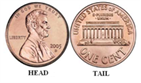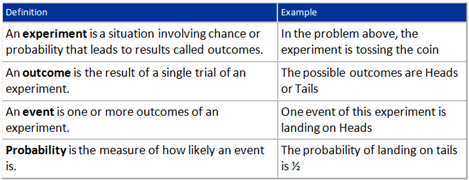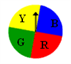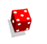# Introduction To Probability

Probability is the likelihood or chance that an event will occur.

If there is no chance that an event will occur, then its probability of occurring will be 0. On the other extreme, if an event is certain to occur, then its probability of occurring will be 100%, or 1. Hence, probability should be a number between 0 and 1, inclusive. But what kind of number?

Suppose your favorite actor has a 1 in 3 chance of winning the Oscar for best actor. This can be measured by forming the fraction 1/3. Hence, a probability is a fraction where the top is the number of ways an event can occur and the bottom is the total number of possible events:

Probability = (Number of ways an event can occur)/(Number of total possible events)

Probability is represented as a pure number p in the range 0 = p = 1

Let us take a typical example to understand the concept of probability Suppose you have a fair coin. It has two sides. One is a head and one is a tailBased on this, we can estimate the probability of getting a result on tossing a coin. i.e., when you toss a coin&#10140; what is the probability of getting heads or what is the probability of getting tails

Let us look at some definitions### Explanation

In the above example; the probability of getting neither heads nor tails on tossing a fair coin is zero; as either heads or tails has to occur

Similarly, the probability of landing on either a head or a tail on tossing a coin is one, or unity; as these two are the only possible outcomes

Let us now look at some more varied examples related to probabilityE.g. 1) A spinner has 4 equal sectors colored yellow, blue, green and red. After spinning the spinner, what is the probability of landing on each color?

Outcomes: The possible outcomes of this experiment are yellow, blue, green, and red. Probabilities of each event can be calculated as follows:

P(yellow) = (number of ways to land on yellow)/(total number of colors) = 1/4

P(blue) = (number of ways to land on blue)/(total number of colors) = 1/4

P (green) = (number of ways to land on green)/(total number of colors) = 1/4

P (red) = (number of ways to land on red)/(total number of colors) = 1/4

Thus, we can arrive at a general formula for the occurrence of an event (A) as

P(A) = (The Number Of Ways Event A Can Occur)/(The Total Number Of Possible Outcomes)

Let us look at another examplee.g. 2) A single 6-sided die is rolled.

(a) What is the probability of each outcome?

(b) What is the probability of rolling an even number?

(c) What is the probability of rolling an odd number?

Suppose you need to calculate the probability of getting 1 on throwing a die.

Let us first look at the possible outcomes of this throw

The possible outcomes of this experiment are 1, 2, 3, 4, 5 and 6, and all outcomes are equally likely

i.e. the probability of getting a “1” is the same as any other number on the die.

Since there are 6 equally likely outcomes in a single throw of a die, there is only one way of getting a particular outcome.Therefore the probability of getting 1 on the die is 1 out of the 6 possible outcomes.

In other words, the probability of getting “1” in a single throw of a die is 1/6 Thus, you can calculate all outcomes as follows

P (even) = (Number of ways to roll an even number)/(total number of sides) = 3/6 = 1/2

P (odd) = (Number of ways to roll an odd number)/(total number of sides) = 3/6 = 1/2

P (1) = (number of ways to roll at 1)/(total number of sides) = 1/6

P (2) = (number of ways to roll at 2)/(total number of sides) = 1/6

P (3) = (number of ways to roll at 3)/(total number of sides) = 1/6 …and so on

Stay tuned with Byju’s to get various lessons and video lectures on different CAT exam topics along with the latest CAT syllabus and pattern. The candidates are also provided with the detailed section-wise explanation and various solved questions for effective preparation.